GeeksforGeeks App
Open AppBrowser
Continue

# Decision Tree Introduction with example

#### A decision tree is a type of supervised learning algorithm that is commonly used in machine learning to model and predict outcomes based on input data. It is a tree-like structure where each internal node represents a decision or test on a specific feature or attribute, each branch represents the outcome of that decision, and each leaf node represents the final decision or prediction.

Here is an example of a decision tree:

Example of a decision tree

Suppose we want to build a decision tree to predict whether a person is likely to buy a new car based on their demographic and behavior data. The decision tree starts with the root node, which represents the entire dataset. The root node splits the dataset based on the “income” attribute. If the person’s income is less than or equal to $50,000, the decision tree follows the left branch, and if the income is greater than$50,000, the decision tree follows the right branch.

The left branch leads to a node that represents the “age” attribute. If the person’s age is less than or equal to 30, the decision tree follows the left branch, and if the age is greater than 30, the decision tree follows the right branch. The right branch leads to a leaf node that predicts that the person is unlikely to buy a new car.

The left branch leads to another node that represents the “education” attribute. If the person’s education level is less than or equal to high school, the decision tree follows the left branch, and if the education level is greater than high school, the decision tree follows the right branch. The left branch leads to a leaf node that predicts that the person is unlikely to buy a new car. The right branch leads to another node that represents the “credit score” attribute. If the person’s credit score is less than or equal to 650, the decision tree follows the left branch, and if the credit score is greater than 650, the decision tree follows the right branch. The left branch leads to a leaf node that predicts that the person is unlikely to buy a new car. The right branch leads to a leaf node that predicts that the person is likely to buy a new car.

In summary, a decision tree is a graphical representation of all the possible outcomes of a decision based on the input data. It is a powerful tool for modeling and predicting outcomes in a wide range of domains, including business, finance, healthcare, and more.

#### Here is an example of a decision tree algorithm:

Begin with the entire dataset as the root node of the decision tree.
Determine the best attribute to split the dataset based on a given criterion, such as information gain or Gini impurity.
Create a new internal node that corresponds to the best attribute and connects it to the root node.
Partition the dataset into subsets based on the values of the best attribute.
Recursively repeat steps 1-4 for each subset until all instances in a given subset belong to the same class or no further splitting is possible.
Assign a leaf node to each subset that contains instances that belong to the same class.
Make predictions based on the decision tree by traversing it from the root node to a leaf node that corresponds to the instance being classified.
For example, let’s say we have a dataset of weather conditions and corresponding activities, and we want to build a decision tree to predict the activity based on the weather conditions. We can use the decision tree algorithm as follows:

Begin with the entire dataset as the root node of the decision tree.
Determine the best attribute to split the dataset based on information gain, which is calculated by the formula: Information gain = Entropy(parent) – [Weighted average] * Entropy(children), where entropy is a measure of impurity or disorder of a set of examples, and the weighted average is based on the number of examples in each child node.
Create a new internal node that corresponds to the best attribute and connects it to the root node. For example, if the best attribute is “outlook” (which can have values “sunny”, “overcast”, or “rainy”), we create a new node labeled “outlook” and connect it to the root node.
Partition the dataset into subsets based on the values of the best attribute. For example, we create three subsets: one for instances where the outlook is “sunny”, one for instances where the outlook is “overcast”, and one for instances where the outlook is “rainy”.
Recursively repeat steps 1-4 for each subset until all instances in a given subset belong to the same class or no further splitting is possible. For example, if the subset of instances where the outlook is “overcast” contains only instances where the activity is “hiking”, we assign a leaf node labeled “hiking” to this subset. If the subset of instances where the outlook is “sunny” is further split based on the humidity attribute, we repeat steps 2-4 for this subset.
Assign a leaf node to each subset that contains instances that belong to the same class. For example, if the subset of instances where the outlook is “rainy” contains only instances where the activity is “stay inside”, we assign a leaf node labeled “stay inside” to this subset.
Make predictions based on the decision tree by traversing it from the root node to a leaf node that corresponds to the instance being classified. For example, if the outlook is “sunny” and the humidity is “high”, we traverse the decision tree by following the “sunny” branch and then the “high humidity” branch, and we end up at a leaf node labeled “swimming”, which is our predicted activity.

Decision tree algorithm falls under the category of supervised learning. They can be used to solve both regression and classification problems. Decision tree uses the tree representation to solve the problem in which each leaf node corresponds to a class label and attributes are represented on the internal node of the tree. We can represent any boolean function on discrete attributes using the decision tree.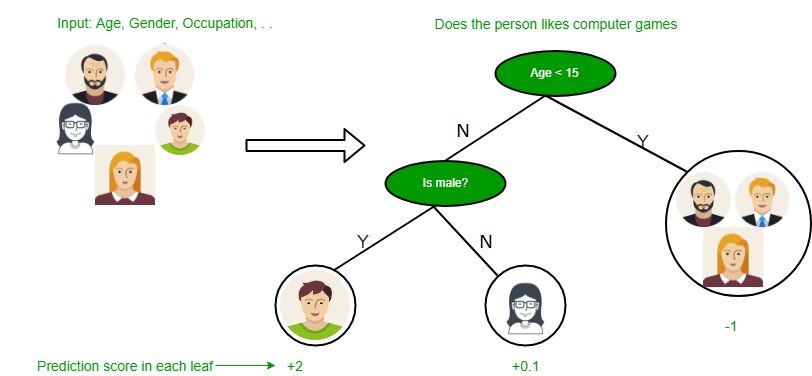Below are some assumptions that we made while using the decision tree:

• At the beginning, we consider the whole training set as the root.
• Feature values are preferred to be categorical. If the values are continuous then they are discretized prior to building the model.
• On the basis of attribute values, records are distributed recursively.
• We use statistical methods for ordering attributes as root or the internal node.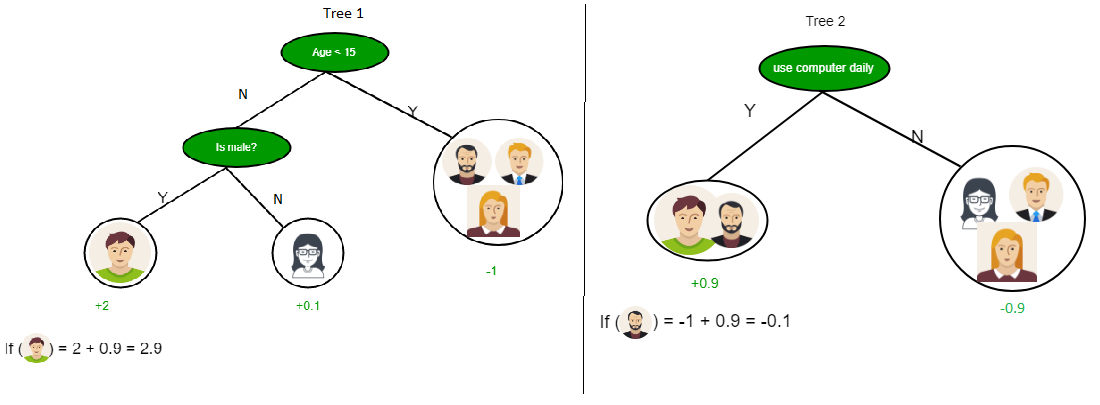As you can see from the above image the Decision Tree works on the Sum of Product form which is also known as Disjunctive Normal Form. In the above image, we are predicting the use of computer in the daily life of people. In the Decision Tree, the major challenge is the identification of the attribute for the root node at each level. This process is known as attribute selection. We have two popular attribute selection measures:

1. Information Gain
2. Gini Index

1. Information Gain When we use a node in a decision tree to partition the training instances into smaller subsets the entropy changes. Information gain is a measure of this change in entropy. Definition: Suppose S is a set of instances, A is an attribute, Sv is the subset of S with A = v, and Values (A) is the set of all possible values of A, thenEntropy Entropy is the measure of uncertainty of a random variable, it characterizes the impurity of an arbitrary collection of examples. The higher the entropy more the information content. Definition: Suppose S is a set of instances, A is an attribute, Sv is the subset of S with A = v, and Values (A) is the set of all possible values of A, thenExample:

For the set X = {a,a,a,b,b,b,b,b}
Total instances: 8
Instances of b: 5
Instances of a: 3
Entropy H(X)  = -\left [ \left ( \frac{3}{8} \right )log_{2}\frac{3}{8} + \left ( \frac{5}{8} \right )log_{2}\frac{5}{8} \right ]

= -[0.375 * (-1.415) + 0.625 * (-0.678)]
= -(-0.53-0.424)
=  0.954

Building Decision Tree using Information Gain The essentials:

• Use info gain to choose which attribute to label each node with
• Note: No root-to-leaf path should contain the same discrete attribute twice
• Recursively construct each subtree on the subset of training instances that would be classified down that path in the tree.
• If all positive or all negative training instances remain, the label that node “yes” or “no” accordingly
• If no attributes remain, label with a majority vote of training instances left at that node
• If no instances remain, label with a majority vote of the parent’s training instances.

Example: Now, let us draw a Decision Tree for the following data using Information gain. Training set: 3 features and 2 classes

Here, we have 3 features and 2 output classes. To build a decision tree using Information gain. We will take each of the features and calculate the information for each feature.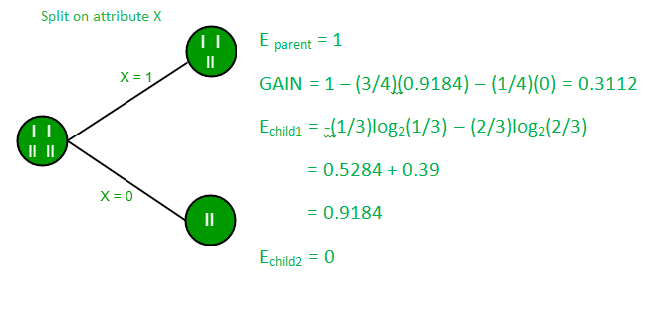Split on feature X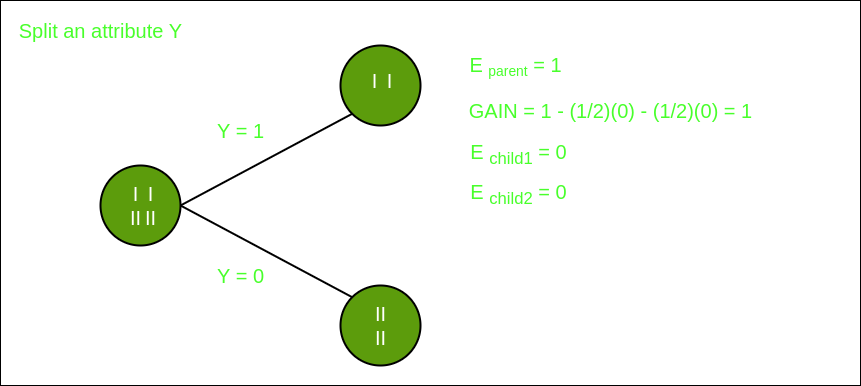Split on feature Y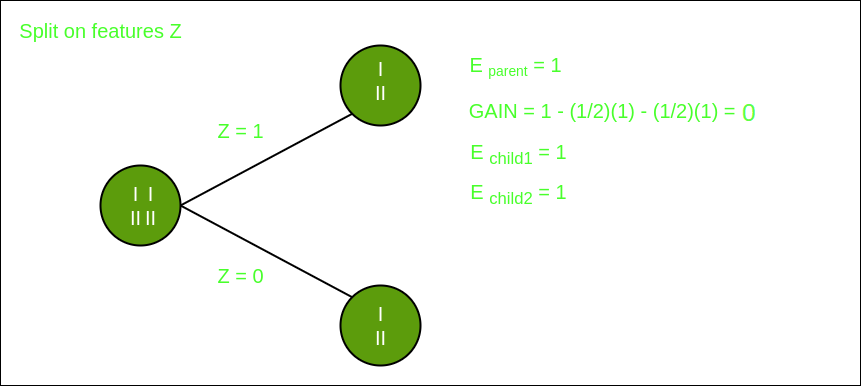Split on feature Z

From the above images, we can see that the information gain is maximum when we make a split on feature Y. So, for the root node best-suited feature is feature Y. Now we can see that while splitting the dataset by feature Y, the child contains a pure subset of the target variable. So we don’t need to further split the dataset. The final tree for the above dataset would look like this: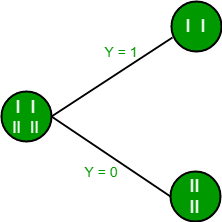2. Gini Index

• Gini Index is a metric to measure how often a randomly chosen element would be incorrectly identified.
• It means an attribute with a lower Gini index should be preferred.
• Sklearn supports “Gini” criteria for Gini Index and by default, it takes “gini” value.
• The Formula for the calculation of the Gini Index is given below.

The Gini Index is a measure of the inequality or impurity of a distribution, commonly used in decision trees and other machine learning algorithms. It ranges from 0 to 1, where 0 represents perfect equality (all values are the same) and 1 represents perfect inequality (all values are different).

#### Some additional features and characteristics of the Gini Index are:

• It is calculated by summing the squared probabilities of each outcome in a distribution and subtracting the result from 1.
• A lower Gini Index indicates a more homogeneous or pure distribution, while a higher Gini Index indicates a more heterogeneous or impure distribution.
• In decision trees, the Gini Index is used to evaluate the quality of a split by measuring the difference between the impurity of the parent node and the weighted impurity of the child nodes.
• Compared to other impurity measures like entropy, the Gini Index is faster to compute and more sensitive to changes in class probabilities.
• One disadvantage of the Gini Index is that it tends to favor splits that create equally sized child nodes, even if they are not optimal for classification accuracy.
• In practice, the choice between using the Gini Index or other impurity measures depends on the specific problem and dataset, and often requires experimentation and tuning

My Personal Notes arrow_drop_up